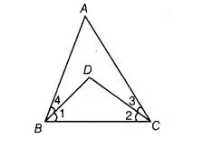`
Question:

In the adjoining figure, we have ∠ABC = ∠ACB and ∠3 = ∠4. Show that BD = DC.Solution:

Given, ∠ABC = ∠ACB …(i)

and ∠4 = ∠3 …(ii)

According to Eulid’s axiom, if equals are subtracted from equals, then remainders are also equal.

On subtracting Eq. (ii) from Eq. (i), we get

∠ABC – ∠4 = ∠ACB  – ∠3 =>∠1 = ∠2

Now, in ABDC, ∠1=∠2

=> DC =BD [sides opposite to equal angles are equal]

BD = DC.